# Numerical Solution for Complex PDE (Ginzburg-Landau Eqn.)

• A
• Johnny_Sparx
In summary, I am looking to numerically solve the Time Domain Ginzburg Landau Equation. I wish to write a python simulator to observe the nucleation of fluxons over a square 2D superconductor domain (eventually 3D, cubic domain). I am using a fourth order Runge Kutta solver for this which I made in Python (the odeint solver does not handle complex variables). How do I implement the RK4 algorithm for complex variables? The equation I am looking to solve is...∂ψ/∂t = -p/i ∇2 - q/i |ψ|

#### Johnny_Sparx

I am looking to numerically solve the (complex) Time Domain Ginzburg Landau Equation. I wish to write a python simulator to observe the nucleation of fluxons over a square 2D superconductor domain (eventually 3D, cubic domain).

I am using a fourth order Runge Kutta solver for this which I made in Python (the odeint solver does not handle complex variables). How do I implement the RK4 algorithm for complex variables?

The equation I am looking to solve is...

∂ψ/∂t = -p/i ∇2 - q/i |ψ|2 ψ + γ ψ

Where p and q are chosen within the complex unit square (unit magnitude), and γ is real on [0,1]. Any help in implementing this would be appreciated. I'd also appreciate if you could point me to software or libraries that can advance my efforts.

First, to clarify, is this what you're trying to solve?

## \frac{\partial \psi}{\partial t} = [-\frac{p}{i} \nabla^{2} - \frac{q}{i} |\psi|^{2}] \psi + \gamma \psi##

Supposing you had a function worked out that could return the right hand side as a function of t, then the Runge-Kutta integration method is the same for a single complex dependent variable as it is for two real dependent variables. You essentially just do Runke-Kutta 4th order integration twice, once for the real part and once for the imaginary part.

If I were going to do this, my first attempt would be to start with a central difference scheme in the 2 space domains and 1 time domain for the special case of q=0, then try to use those as trial functions, maybe adding in some correction functions based on intuition/hunch about what q does to the solutions or just observational insight. There are probably more elegant and effective methods.

Hope that helps!

Yes, it does help. Your equation is the one I want to solve, it seems I could not render my equation correctly (new to BB codes). When I think about it, there are no restrictions on the RK algorithm (ie. no restriction to stay with the real domain). Given that Python can handle complex numbers, I wonder why I have to modify my direct RK implementation. Perhaps there is something within NumPy that makes this an issue.

I will try this now, but need a predictable test case to see if things are working out, something non-trivial but straightforward to test the RK4 algorithm. If you have a simple suggestion - I'd appreciate it. By the way, how did you render your equation? My BB coding was not as legible as yours.

For a test case, try something simple like ##\frac{dz}{dt} = -9.81it##. Then try ##\frac{dz}{dt} = -9.81t##. Then try something like ##\frac{dz}{dt} = iz##.

As for the fractions, \frac{x}{y} => ##\frac{x}{y}##

I'm using Python/NumPy to do the simulation and it has complex variable handling. The RK4 algorithm does not seem to have restrictions on domain, but I tried full separation of real and complex parts as you suggested. Furthermore, I appreciate the simple test cases you suggested and used them.

The fully separated version seemed to match the output from the plain/direct implementation but I needed special handling to take care of basic 1 and 2D ODEs which I did (I'm trying to create a 2D simulation for now). Here's another question: How to implement a complex Laplacian? The TDGL equation has ∇2 ψ. At the moment, I used a discrete convolutional kernel to find the Laplacian of a purely real 2D ψ array. Should the same kernel be used for complex values?

See: https://en.wikipedia.org/wiki/Discrete_Laplace_operator#Finite_Element_Method

I am really having trouble with this simulation and do appreciate help.

Johnny_Sparx said:
Here's another question: How to implement a complex Laplacian? The TDGL equation has ∇2 ψ. At the moment, I used a discrete convolutional kernel to find the Laplacian of a purely real 2D ψ array. Should the same kernel be used for complex values?

See: https://en.wikipedia.org/wiki/Discrete_Laplace_operator#Finite_Element_Method

I am really having trouble with this simulation and do appreciate help.

You can use the discrete Laplacian on the real part and imaginary part separately. This isn't a huge issue. What's going to be hard is the nonlinear term in the PDE. I don't think you'll be able to write a discrete matrix operator for it like you do for the Laplacian, since that term has 3 powers of psi in it. This is where I think you may need to start turning to trial functions or a finite element analysis as opposed to a finite difference analysis. One idea that comes to mind is solving the problem for q = 0 via the finite difference approach and then using trial functions (kind of like a Galerkin method) to find the right correction to account for the q-dependent term. Beyond that, I don't have enough experience to make recommendations. Anyone more familiar with numerical strategies for this equation?

I can't seem to embed an image, but I did a test on a 2D domain: \frac{dψ}{dt} = ∇ψ:

*** 0.00s to 50.00s in 100 timesteps.
*** -10.00 < x < 10.00, delta_x = 200
*** -10.00 < y < 10.00, delta_y = 200
*** Starting simulation at 2016-06-30 15:51:20.
*** Running ODE solver in array mode.
*** Simulation concluded after 9 uS.

The initial value was a 2D step function = 1.0+1.0j between +/- 4 in both axes. My software ran without crashing (a good thing), and the output is roughly what I'd expect it to be at the end of simulation time. Our conversation helped clear things up in that sense.

http://www.massey.ac.nz/~dpplayne/Papers/cstn-070.pdf
1. In this report, Equation 7 sticks out. The first term on the right hand side makes use of the 1D Laplacian with no issues. For each step in the RK algorithm, ψ(x,t) has t held constant so the Laplacian over the complex field should pose no problem. The partial derivatives should be fine.
2. The second term throws me off somewhat, but |ψ|2 ψ seems to simply a complex scalar field... am I missing something here?
I think I am likely missing some very basic insight into how the GL equation should be computed. If there were a Python package that solved this for me, I'd be ecstatic but looks like there's only something for the London Equations (because they are restricted versions of Maxwell's equations which can be solved using the FDTD method and there's lots for that).

How important is system size? If not so much how about a super simple exponential time integration scheme in Fourier space with some simple(or no) anti-aliasing on the non-linear term? I seem to recall it worked pretty well back when I worked with it. Have you read The world of the complex Ginzburg-Landau equation by Aranson and Kramer? They are bound to have some references to numerical schemes.

I will have to review what you suggested in detail, I think I have seen this reference somewhere. Furthermore, I am not familiar with the method you speak so it will be worthwhile to look at this.

At the moment, I am trying to push forward with what I have... I don't know how to upload a video to PF, but i think I got something that make sense to a neophyte in superconductivity (my background is in electrical and computer engineering). I randomly initialized a complex field (the order parameter). Over time, the system seems to demonstrate fluxon nucleation... this is to say, normal cores in a sea of superconducting fluid.

Some updates for Twigg and now you Strum...
1. The fluxon cores do not seem to move. I assume this is because they have enough space not to demonstrate their inter-repulsive forces.
2. The cores seem to have a very low concentration of superfluid, consistent with GL theory.
3. The simulation seems to overflow at times... I suspect this has to do with the 5-point stencil I am using to compute the Laplacian. The convolutional kernel I am using does not divide by 4, nor does it take into account the spatial resolution of the simulation (ie. h)
4. The simulation runs slow. I may need to move over to a GPU to do the computations, I want to do this in 3D.
5. I can generate images and video that look pretty, but that doesn't count for anything technical. I somehow need to validate this thing.
So now, I am looking for two things in the short term as I review the suggested doc...
1. Getting my Laplacian to work more numerically accurately and not produce overflows.
2. Find some sort of validation strategy.
3. Buy an new laptop with a GPU.#### Attachments

•2D_GL_Equation_Simulation_2_06JUL2016.png
103.7 KB · Views: 989
Before you start shopping new computers, is the code you're using to take the Laplacian your own original source code? If so, did you use sparse matrix encoding? I have never had an issue with a Laplacian matrix being too big for the processor to handle, even in 3D, while using sparse matrix encoding. Granted, it could still be that you are asking for higher resolution than I have ever attempted. But I was able to solve Poisson's equation on a MacBook Pro from 2011 using this approach with fifty to a hundred nodes per dimension. A friend of mine was able to take this simulation code and put it in a loop to simulate tens of different geometries and display the results as a real time movie, without buffering the simulation data. I have a hard time believing the Laplacian matrix itself is your biggest demand on memory. Can you verify this?

I'm unfamiliar with the physics involved, so I'll have to review these papers before I make any further comments. I just figured you might want the above information before you invest in a new laptop.

It looks like MATLAB colormap. In MATLAB depending on your implementation you do not need a matrix formulation. You can directly vectorize the input. It is a bit hard to see the lattice size but already 512^3 needs some memory.

For validation, perhaps you can look at the velocity statistics of the vortices( which I by the way recall as moving, either anihilating or repelling. Perhaps the friction coefficient can make them stationary?)? You could look at this paper:
Anisotropic velocity statistics of topological defects under shear flow, http://arxiv.org/pdf/1201.5758v1.pdf
which have a few results and also describes a really nice method for calculating the charge and velocity of the vortices.

For the numerical method I suggested, I can give you a fast description later if you like.

Also if you do not mind I would like to see your Runge-Kutta code. I never got around to really learn this method despite it being all over the place.

Twigg said:
Before you start shopping new computers, is the code you're using to take the Laplacian your own original source code? If so, did you use sparse matrix encoding?
Yes it is, and no I did not use sparse encoding as I did not think it was necessary here, the ψ(x,y) (order parameter) matrix is a scalar field.

Twigg said:
I have never had an issue with a Laplacian matrix being too big for the processor to handle, even in 3D, while using sparse matrix encoding. Granted, it could still be that you are asking for higher resolution than I have ever attempted. But I was able to solve Poisson's equation on a MacBook Pro from 2011 using this approach with fifty to a hundred nodes per dimension. A friend of mine was able to take this simulation code and put it in a loop to simulate tens of different geometries and display the results as a real time movie, without buffering the simulation data. I have a hard time believing the Laplacian matrix itself is your biggest demand on memory. Can you verify this?
I implemented the discrete Laplacian operator using a convolutional kernel for my 2D complex scalar field and this is an O(n2) complexity operation. The Runge-Kutta integration itself has linear complexity but is applied over the 2D field. This can get computationally intensive, but not necessarily memory intensive IMHO (there is very little internal buffering for the actual computation). I did some very basic profiling and realized that the graphic rendering is what is the memory hog. My poor laptop. After a bit of rendering... the fan goes crazy... This week, I will be validating the Laplacian with a known discretized analytic function. I will work things out on paper and then inspect the numerical result. I will be sure to get back to you on this.

Twigg said:
I'm unfamiliar with the physics involved, so I'll have to review these papers before I make any further comments. I just figured you might want the above information before you invest in a new laptop.

To be honest, my computer is an old dual core unit that is filled with software used at my different jobs over the past 6+ years. Disk/memory swapping may be my problem, but regardless - this PC needs to be replaced soon... I just need a catalyst. I am looking at a MSI gaming laptop that will give me some CUDA capability. I simply can't wait for 5 minutes between simulation trials as I am testing and things should get more complex and this will cumulate to the point of effective work stoppage. My PhD is a part time affair as I have two full time jobs... sleep is an important scheduled activity. This was actually at the forefront of my thoughts when browsing for laptops. My circumstance is not one of choice.Strum said:
Also if you do not mind I would like to see your Runge-Kutta code. I never got around to really learn this method despite it being all over the place.
Is there a way I can paste Python code in this forum? I seem to not be very good at figuring out how to perform basic posting operation. I absolutely don't mind sharing the code. It is basic, but works well for 1D and 2D fields with no modification. With basic modification, you can specify functional/control systems using state equations... that is REALLY cool for lumped physical models. I just tried cutting and pasting my code: hideous.

I wanted to make a video of my work in progress, although it is not completely validated and bug free. I put up a temporary YouTube video to share what I have at the moment:

I will still be validating the Laplacian later on among other things.

Very cinematic! What is your unit of length in the simulation box?

Perhaps you can paste your code using the code tags.

Code:
test

Okay... seems like my implementation of the Laplacian has some performance issues.My validation method:
1. Used a 2D function and computed the symbolic Laplacian so I had a perfect reference: f(x,y) = exp(-x2-y2). This is a single 2D hump centered at (0,0). Most of it vanishes at a distance of 2 (radial) units from this center.
2. Set up the discrete Laplacian function: 5-point stencil & convolution.
3. For various domain extents (the range of x and y), I computed the error between the analytic and discrete solutions.
4. Generated error plots for each range, with varied values for h (the finite difference step).
5. BONUS: A psuedocolor plot of the surface as well as the error surface resulting from the aforementioned comparison.
Attached are the results (plots are annotated).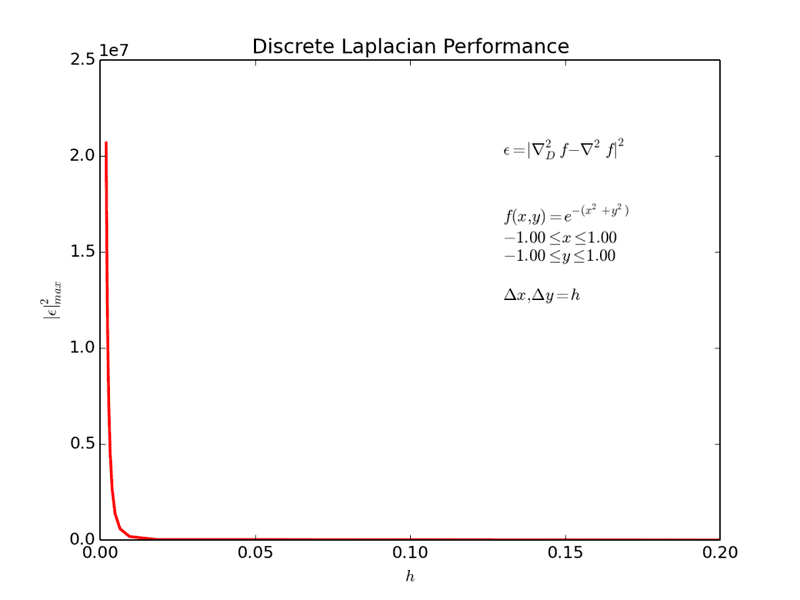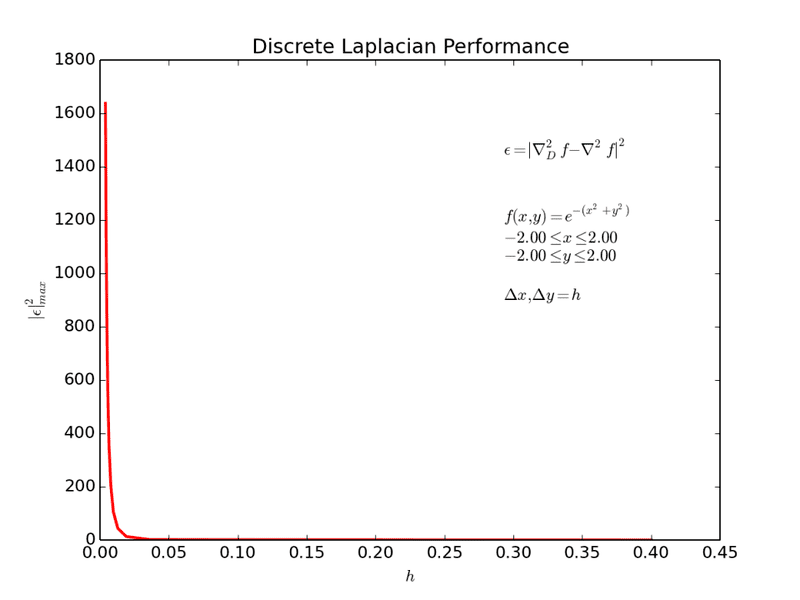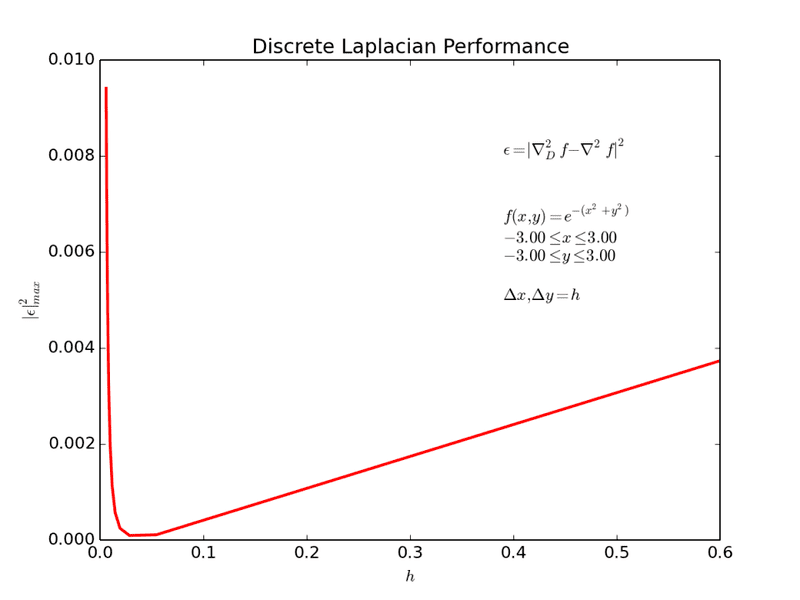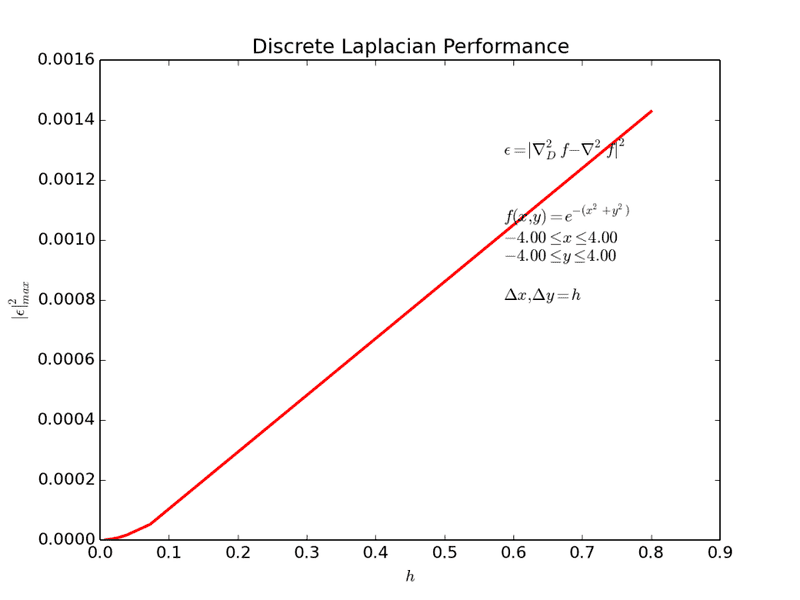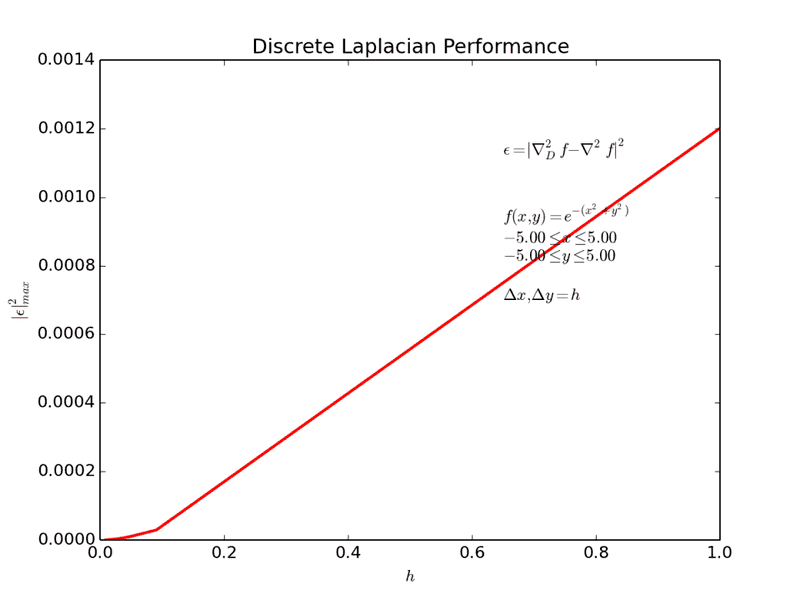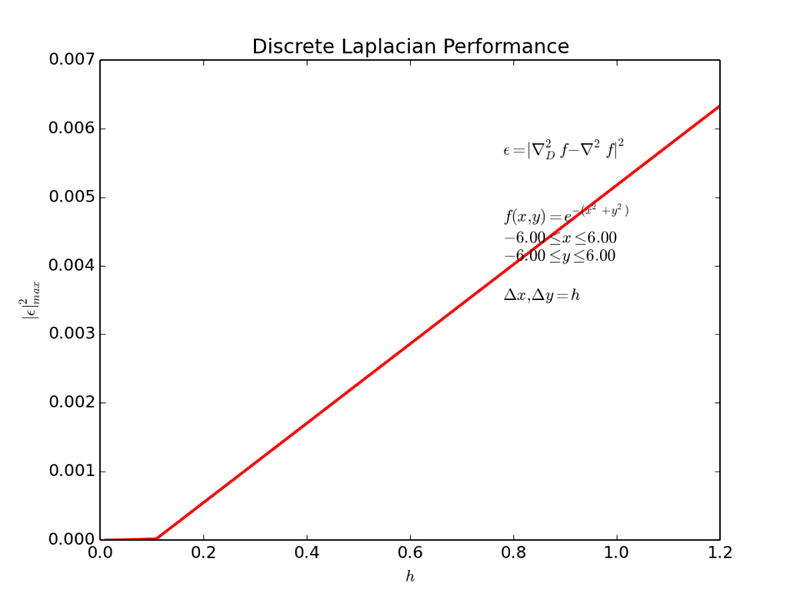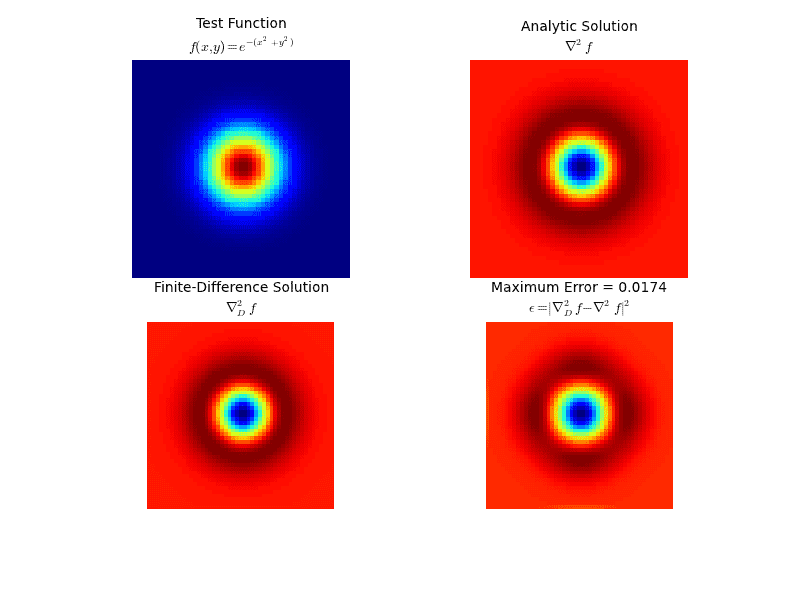Now this was an INTERESTING exercise. The maximum error that results can be dramatic. As the domain extents come closer to the hump's center (the domain region clips the hump. This is to say, the function is not allowed to vanish. Edge effects take a serious toll here. This is what is likely causing my overflow problems. As the domain is extended further away from the center, the function begins to vanish and the error vanishes as well.

I suppose, my question is... is my convolution method just a quick and dirty method of computing the function Laplacian or is there a better way. I know Twigg mentioned a method that involved sparse matrices. I did some thinking while driving and tried to figure out why he mentioned this. Now I can see this as another method (compute the finite difference at each point on the vectorized surface and solve the resulting system)... Can I get more information on this, like an algorithm name?

Code rendering on this forum is terrible/non-existant. A tab character won't render (Python loves tabs), if there is another solution for posting code - I wouldn't mind knowing about it.

•Twigg
Strum said:
Very cinematic! What is your unit of length in the simulation box?

Perhaps you can paste your code using the code tags.

Code:
test

Goodness, the code tags don't like tabs. This ruins any formatting that makes the code legible! My simulation length is kinda generated from random parameters (yes, I know... I'm testing stuff).

I made the video kinda cinematic deliberately. When I work in industry, you need to get the attention of your peers in R&D. This works. So I just fall back to old habits. Some of my technical videos look like there should be a rapper in there somewhere. It gets the point across in a pleasant way and costs little time. I bring this habit to academia now...Strum said:
Also if you do not mind I would like to see your Runge-Kutta code. I never got around to really learn this method despite it being all over the place.
This is not an ideal posting, but I could not ignore your request despite the forum's poor rendering ability (AFAIK).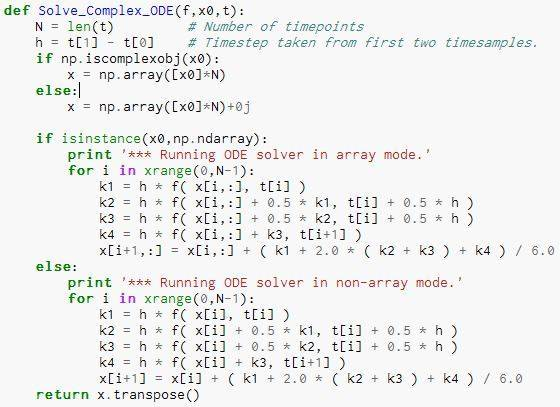This will work for 1D or 2D functions (the second block is straight from the textbook for simple ODEs). With some modification, you can use it to solve state-space equations. If you do any engineering, you can simulate some pretty cool systems. I actually implemented this code on a microcontroller and it worked. I had a real-time thermal simulator. I could heat or cool a virtual piece of material arbitrarily and monitor its temperature. I found it to be pretty impressive, but coupled ODEs are even cooler.

Johnny_Sparx said:
I suppose, my question is... is my convolution method just a quick and dirty method of computing the function Laplacian or is there a better way. I know Twigg mentioned a method that involved sparse matrices. I did some thinking while driving and tried to figure out why he mentioned this. Now I can see this as another method (compute the finite difference at each point on the vectorized surface and solve the resulting system)... Can I get more information on this, like an algorithm name?

I thought this was what you were doing all along. Sorry for misunderstanding! I don't know the fancy name for it, but what I did is I constructed a 3D discrete Laplacian sparse matrix using kronecker products. There's a formula for that on wikipedia. For example, if I had a 2D 3x3 mesh, I would ordinarily need a 9x9 discrete Laplacian matrix with 81 entries. Using sparse encoding, you cut that number down to only the nonzero entries, which are only a few diagonals, and save a lot of memory. I think the math is the same as the convolutional kernel you are describing. It speeds things up a lot.

Johnny_Sparx said:
Now this was an INTERESTING exercise. The maximum error that results can be dramatic. As the domain extents come closer to the hump's center (the domain region clips the hump. This is to say, the function is not allowed to vanish. Edge effects take a serious toll here. This is what is likely causing my overflow problems. As the domain is extended further away from the center, the function begins to vanish and the error vanishes as well.

I have a speculative theory on this, hopefully it is productive. This convolutional kernel business doesn't seem to take spatial boundary conditions into account. I'm basing this off my experience with the discrete Laplacian method I described above (which, if you think about it, uses the same principle) and Jackson's treatment of Green's functions. The convolution matrix method to me seems like taking an integral convolution, which is like taking the convolution of a Green's function with a source distribution. Green's functions depend on the boundary conditions (explained fully in Jackson, or just take an image charge as an example). For some more concrete connection here, when I used the discrete Laplacian matrix method (which I _think_ is mathematically identical to the convolutional kernel), we had to delete all entries for mesh points on the boundaries, edges, and corners of our rectangular grid and insert driven constraints from physical considerations. It doesn't give you the usual discrete Laplacian when you consider boundary conditions (Dirichlet or von Neumann, either one changes stuff up). In the case of Dirichlet conditions, I would replace an entire row of the Laplacian with a row consisting of a single '1' and all others '0's, and put the corresponding data point in the solution vector. The problem I was working on was a heat transfer problem, so I didn't have any true von Neumann conditions to work with, but for boundary heat sources I had to use a modified row for the discrete Laplacian, which I figured out a pattern for by doing simple examples by hand, plus the heat source coming in from the outside. I don't know if these simplified boundary conditions are helpful for your application, but I have a hunch that boundary conditions are what's causing your edge-related errors.

NOTE: The discrete Laplacian formula from wikipedia doesn't include boundary effects either! I had to derive a pattern/strategy by doing very small cases by hand.

I don't think I made this very clear in my last post. The ordinary discrete Laplace operator that's described in the paper you posted works for any point on the interior of your domain. In the presence of boundary conditions, you modify the operator on points on the boundary accordingly. The interior point Laplacian operator expects for every mesh cell that it is surrounded by six other mesh cells. I suspect that your overflow errors are due to the Laplacian "looking" for mesh cells that aren't there because of the finite extent, if that makes sense.

@Johnny
A max error on the laplacian of around 0.005 for even large dx for this function seems pretty good to me. On the other hand in your 2d plots it looks weird that the error does not go towards zero away from the middle( assuming red is not = 0. You should put in a colorbar on those plots ). My own fast implementation of both 5 and 9 point stencils goes to zero pretty quickly,
Thank you for the code, I will look at it perhaps sunday. :)

I can't seem to figure out how to edit the post :/. Here is a pic of the error I get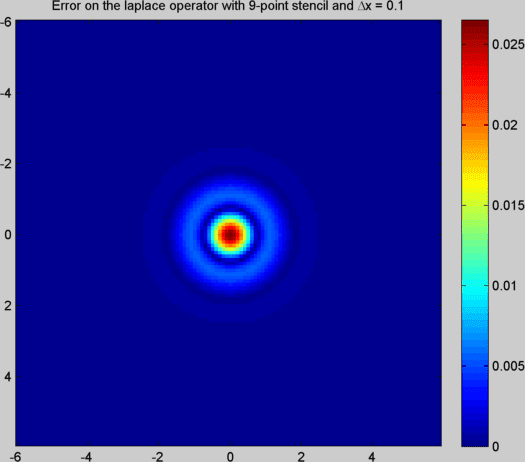http://tinypic.com/r/25tjkm1/9

Twigg said:
I don't think I made this very clear in my last post. The ordinary discrete Laplace operator that's described in the paper you posted works for any point on the interior of your domain. In the presence of boundary conditions, you modify the operator on points on the boundary accordingly. The interior point Laplacian operator expects for every mesh cell that it is surrounded by six other mesh cells. I suspect that your overflow errors are due to the Laplacian "looking" for mesh cells that aren't there because of the finite extent, if that makes sense.

I needed to take some time to think about this whole thing. The convolution method I was working with has a spectral response. By this I mean mean the finite boundary extents should yield oscillations at the extents, and these are related to the spatial step size. When I initialize my array with uniformly random values (from the complex unit square with length less than or equal to one), relative to one another, these are all 2D step functions. For example, for an arbitrary array location (i,j) assume I have a random value of 1+0j. Adjacent to it, say at (i+1,j-1) there may be a value of 0+0j. This is a sharp discontinuity whose second derivative is quite large.

For a sufficiently large initialization array with values that are locally discontinuous the Laplacian can yield large values, especially the way I am iterating for the Runge-Kutta algorithm... repeatedly calling the Laplacian operator. I am just throwing ideas around... what do you think?

Strum said:
I can't seem to figure out how to edit the post :/. Here is a pic of the error I get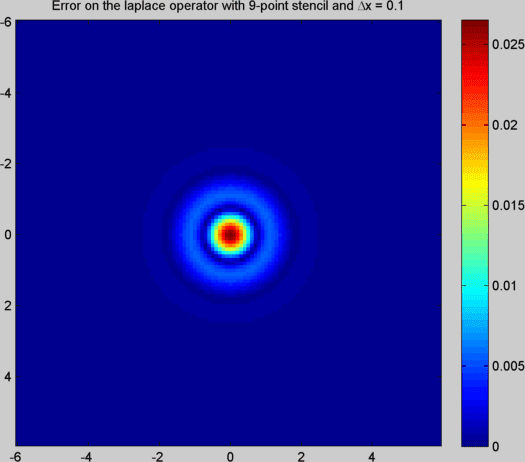http://tinypic.com/r/25tjkm1/9
(How did you get your pic to appear inline?)

I notice you are using a 9-point stencil here, while I was using a 5-point stencil (on the rectangular grid, you are taking diagonals into account while I am not). You were also using convolution here?

Strum said:
I can't seem to figure out how to edit the post :/. Here is a pic of the error I gethttp://tinypic.com/r/25tjkm1/9

Strum, without knowing the exact 9-point stencil you used I tried to replicate the plot you produced (in Matlab I assume?). Apparently, there is no standard for the 9-point kernel although there is some consensus on the 5-point kernel. Different formulations of the finite difference formulae will yield differing kernels. So when I use a 9 point kernel, I obtain the following for the same domain and $\Delta x = \Delta y = h = 0.1$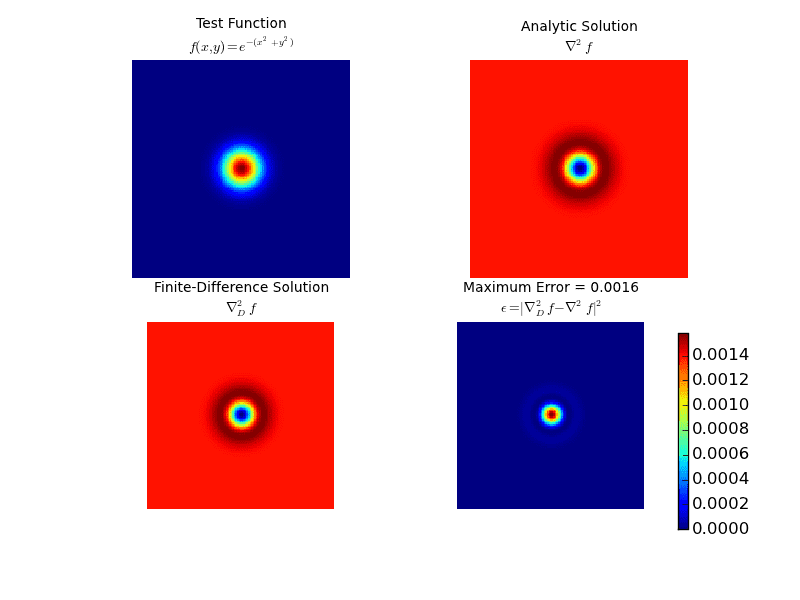It seems like I get a very small $|\epsilon|^2$By the way, the reason I am insisting on using convolution is purely for computational reasons: it can be done in hardware (ie. GPU). It seems like we both have comparable rates. The 5-point stencil yields the following result: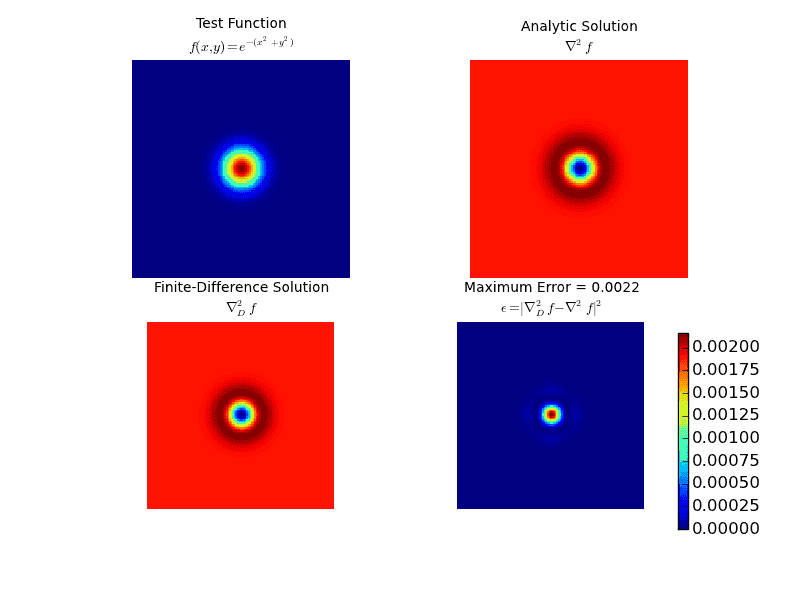Notice that there are some artefacts that appear about one of the "color rings" in the last errror plot. Were you using another method other than convolution?

First off, I want to say thanks to Johnny_Sparx for taking the time to make this a great thread. I've learned a lot from it and enjoyed reading every new post.

Johnny_Sparx said:
I needed to take some time to think about this whole thing. The convolution method I was working with has a spectral response. By this I mean mean the finite boundary extents should yield oscillations at the extents, and these are related to the spatial step size. When I initialize my array with uniformly random values (from the complex unit square with length less than or equal to one), relative to one another, these are all 2D step functions. For example, for an arbitrary array location (i,j) assume I have a random value of 1+0j. Adjacent to it, say at (i+1,j-1) there may be a value of 0+0j. This is a sharp discontinuity whose second derivative is quite large.

For a sufficiently large initialization array with values that are locally discontinuous the Laplacian can yield large values, especially the way I am iterating for the Runge-Kutta algorithm... repeatedly calling the Laplacian operator. I am just throwing ideas around... what do you think?

I may be missing something, but I don't see how the oscillations at the extents are related to the error in the Laplacian due to large differences divided by small step sizes. There is a way you could test to see if these differences are what's giving you the overflow. Go into your initialization code where you sample random points from the complex unit square and make the radius of the complex square a variable, not necessarily 1. For clarity, let's call the size of the complex square that the initial values are sampled from g, and let's call the stepsize h. Write a for loop to run your simulation for several values of g and h. My instinct would be to have it do orders of magnitude. For example, the loop would start with h = 1.0E-03 and g = 1.0E-03 and run your simulation, then do h = 1.0E-03 and g = 1.0E-02, etc., then h = 1.0E-3 and g = 1.0E3, then h = 1.0E-02 and g = 1.0E-03, etc etc. If you are right and the issue is entirely due to large differences in the Laplacian, then the overflow error will only depend on g/h. If you plot the overflow error over the gh-plane, the resulting surface plot should be independent of the radial coordinate and depend only on the angular coordinate (on average, at least, since the initialization is randomized). If that's not the case, I would venture to say that something else is up. This may take a lot of computing time.

I maybe should've asked a little earlier, but can you tell us what you're using as boundary conditions, and how you tell the kernel to behave on those boundaries? For example, on the edges are you using a 4-point stencil or is there some contribution from outside? I can't tell if the errors you're seeing are related to boundaries or not. Hopefully the above test helps narrow it down.

Twigg said:
First off, I want to say thanks to Johnny_Sparx for taking the time to make this a great thread. I've learned a lot from it and enjoyed reading every new post.
Truth be known, in my personal circles, I have been talking about the direction both you and Strum have helped me with. I just posted, you both replied. And I too have been learning a lot of things. Lots of deep insight in this thread. Thought provoking stuff!

Twigg said:
I may be missing something, but I don't see how the oscillations at the extents are related to the error in the Laplacian due to large differences divided by small step sizes.
My inspiration for saying this extends from the following idea:
Consider a unit step function $f(t)$ (ie. Heaviside function with one dimensional discontinuity). The 1D laplacian of this function is essentially the second derivative $\frac{\partial^2}{\partial t^2}f(t)$. This is the doublet function, a double sided, unit impulse (FYI, the Kroniker delta function is a discrete version of the Dirac function and the doublet is its derivative). In the frequency domain these impulses have an infinitely wideband spectrum (constant in the frequency domain). Discretely sampling an infinitely wideband signal essentially filters it. To me, derivatives at crisp boundaries creates time domain oscillations that are band-limited by the sampling frequency. That's why when we look at simulations involving Laplacians or gradients over time, amplitude changes are typically initiated at boundaries that are sharp and have wave-like patterns.
I just wanted to express motivations for my comment, thanks for calling me to task for this. It helped me clarify my thoughts on the matter.

Twigg said:
There is a way you could test to see if these differences are what's giving you the overflow. Go into your initialization code where you sample random points from the complex unit square and make the radius of the complex square a variable, not necessarily 1. For clarity, let's call the size of the complex square that the initial values are sampled from g, and let's call the stepsize h. Write a for loop to run your simulation for several values of g and h. My instinct would be to have it do orders of magnitude. For example, the loop would start with h = 1.0E-03 and g = 1.0E-03 and run your simulation, then do h = 1.0E-03 and g = 1.0E-02, etc., then h = 1.0E-3 and g = 1.0E3, then h = 1.0E-02 and g = 1.0E-03, etc etc. If you are right and the issue is entirely due to large differences in the Laplacian, then the overflow error will only depend on g/h. If you plot the overflow error over the gh-plane, the resulting surface plot should be independent of the radial coordinate and depend only on the angular coordinate (on average, at least, since the initialization is randomized). If that's not the case, I would venture to say that something else is up. This may take a lot of computing time.
This might take some time to do which I don't think I have. What I did do was to test the Laplacian I implemented with a function that did not vanish at the borders. In other words, I used the same function, but biased it by 1 and computed the Laplacian with 0 as fill values at the border. Essentially this forms a crisp edge. The function used was:$$f(x,y) = {{\rm e}^{-{x}^{2}-{y}^{2}}}+1$$ Note the last term. The analytic Laplacian was found to be:$$\nabla^2 f = -4\,{{\rm e}^{-{x}^{2}-{y}^{2}}}+4\,{x}^{2}{{\rm e}^{-{x}^{2}-{y}^{2}}}+4\,{y}^{2}{{\rm e}^{-{x}^{2}-{y}^{2}}}$$
The 9-point stencil was convolved over the discretized function ($-6 \leq x,y\leq 6, h=0.1$). The convolutional function was called with fill values of 0 at the boundaries. The result: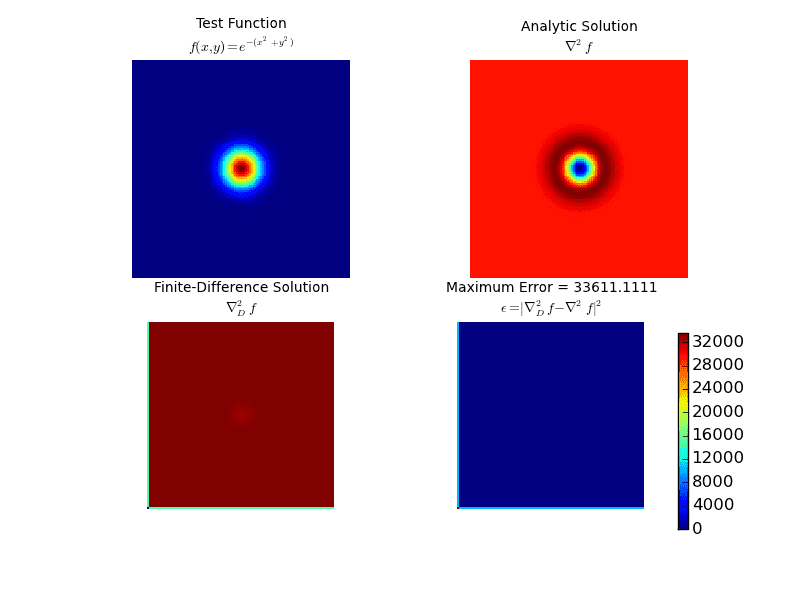And look at those errors! 6 orders of magnitude greater than the previous test result I posted. It seems the convolutional method does not respect boundaries as expected. Next experiment. Convolution using a "wrap" at the end of both axes, ie, the domain is a torus. This continuous domain yields (for the same function):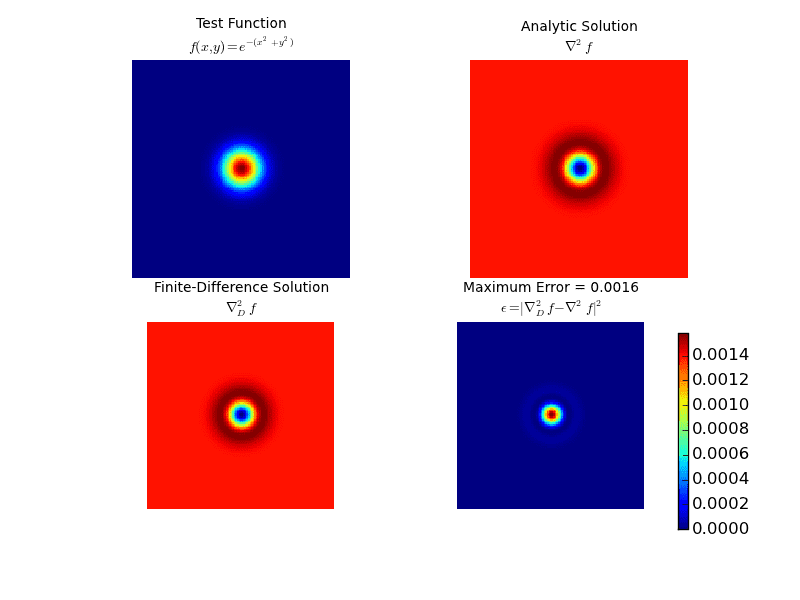And now look at the boundary. My conclusion seems to be converging to:

In computing a discrete Laplacian, it seems convolution with a 5/9 point stencil (or any other) works on continuous boundaries - not on discrete ones, despite what fill values you insert at the boundaries.

Twigg said:
I maybe should've asked a little earlier, but can you tell us what you're using as boundary conditions, and how you tell the kernel to behave on those boundaries? For example, on the edges are you using a 4-point stencil or is there some contribution from outside? I can't tell if the errors you're seeing are related to boundaries or not. Hopefully the above test helps narrow it down.

At the boundary, I need for the order parameter to be zero, ie $\psi=0$ at the boundaries. What is the name of a method I can use that does not employ convolution to enforce these boundaries? I know we mentioned the use of sparse matrices... is there a name for this method so that I can look it up?

Johnny_Sparx said:
Were you using another method other than convolution?
I used a simple 5 or 9 point finite difference stensil.

You result in #28 is to be expected since you are differentiating a function given by ## f(x,y) = e^{-(x^2 + y^2)} + 1 ##, in the interior and ## f(x,u) = 0 ## on the boundary. That will generate problems. This however, is not the situation you will be in when doing your simulation, where the G-L field will find a natural solution with ## \psi = 0 ## on the boundary.

Johnny_Sparx said:
In computing a discrete Laplacian, it seems convolution with a 5/9 point stencil (or any other) works on continuous boundaries - not on discrete ones, despite what fill values you insert at the boundaries.
I am not entirely certain I understand what you are saying but I am suspecting it does not make sense. There is no problem specifying Dirichlet boundary conditions using all sorts of different numerical methods.

Johnny_Sparx said:
At the boundary, I need for the order parameter to be zero, ie ## \psi=0 ## at the boundaries. What is the name of a method I can use that does not employ convolution to enforce these boundaries? I know we mentioned the use of sparse matrices... is there a name for this method so that I can look it up?

Perhaps you should try and use a finite difference method. See for example these slides: http://people.sc.fsu.edu/~jpeterson/notes_fd.pdf . In order to enforce your boundary conditions you extend the lattice with a boundary layer with a specific value( in your case ## 0 ## ), which you do not update. This is shown on slide 16. On slide 18 the differentiation matrix for a finite difference method is shown. As you can see it is sparse.

Finite difference is the method I was thinking of. Specifically, a central-difference scheme for the Laplacian. The catch is that your problem is non-linear due to the ##|\psi|^{2} \psi## term. You will need to use Newton's method to get a solution, and that opens a new can of worms with convergence and validation. I've never tried this personally, but I know at least one piece of commercial software (COMSOL) that uses Newton's method to solve the matrix problems of finite element methods.

Is there a way to adapt your existing code to initialize the order parameter on the boundaries to 0 and then tell the convolutional kernel to act on everything but the outermost cells? That might make a lot more sense if you already have a method that works. For example, if your initial data was [0, 0, 0, 0; 0, 0.3+0.5i, 0.5-0.2i,0;0,0,0,0], then you would tell the convolutional kernel only to operate on the inner two entries and not act on the boundary zeros. Is that possible? Just a thought.

Twigg said:
Finite difference is the method I was thinking of. Specifically, a central-difference scheme for the Laplacian. The catch is that your problem is non-linear due to the |ψ|2ψ|\psi|^{2} \psi term. You will need to use Newton's method to get a solution, and that opens a new can of worms with convergence and validation. I've never tried this personally, but I know at least one piece of commercial software (COMSOL) that uses Newton's method to solve the matrix problems of finite element methods.

Why would you think there is a problem with non-linear terms? I really can not see how this should pose a problem as long as he uses some explicit time integration scheme ( and even if he used an implicit I can not see why the difficulties would even be related to the finite difference method ).

Strum said:
Why would you think there is a problem with non-linear terms? I really can not see how this should pose a problem as long as he uses some explicit time integration scheme ( and even if he used an implicit I can not see why the difficulties would even be related to the finite difference method ).
You're absolutely right, my mistake. I was thinking of a steady-state problem, which this is not.